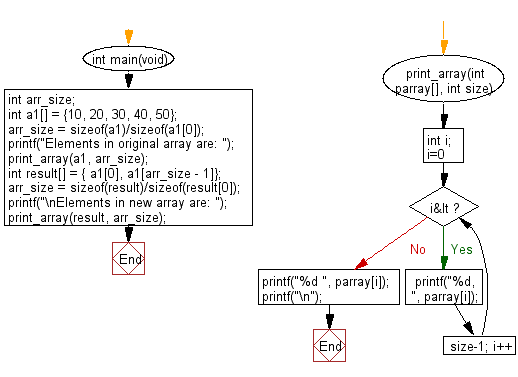﻿ C : Array based on the first and last elements of an array# C Exercises: Create a new array taking the first and last elements of a given array of integers and length one or more

## C-programming basic algorithm: Exercise-40 with Solution

Write a C program to create a new array taking the first and last elements of a given array of integers and length one or more.

C Code:

``````#include <stdio.h>
#include <stdlib.h>
int main(void){
int arr_size;
int a1[] = {10, 20, 30, 40, 50};
arr_size = sizeof(a1)/sizeof(a1);
printf("Elements in original array are: ");
print_array(a1, arr_size);
int result[] = { a1, a1[arr_size - 1]};
arr_size = sizeof(result)/sizeof(result);
printf("\nElements in new array are: ");
print_array(result, arr_size);
}
print_array(int parray[], int size)
{
int i;
for( i=0; i<size-1; i++)
{
printf("%d, ", parray[i]);
}
printf("%d ", parray[i]);
printf("\n");
}
``````

Sample Output:

```Elements in original array are: 10, 20, 30, 40, 50

Elements in new array are: 10, 50
```

Pictorial Presentation:Flowchart:C Programming Code Editor:

What is the difficulty level of this exercise?

Test your Programming skills with w3resource's quiz.

﻿